# Remote Sensing Images Semantic Segmentation with General Remote Sensing Vision Model via a Self-Supervised Contrastive Learning Method

## 基本信息

\1.标题：Remote Sensing Images Semantic Segmentation with General Remote Sensing Vision Model via a Self-Supervised Contrastive Learning Method

\2.作者：Haifeng Li, Yi Li, Guo Zhang, Ruoyun Liu, Haozhe Huang, Qing Zhu, Chao Tao

\3.作者单位：Central South University

\4.发表期刊/会议：arXiv

\5.发表时间：2021

\6.原文链接：https://arxiv.org/abs/2106.10605

## 1.Introduction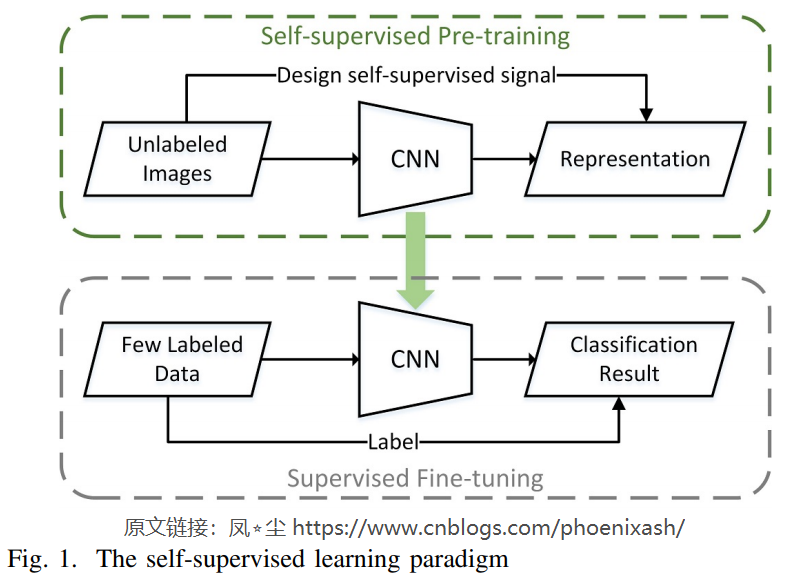​ 1) 据我们所知，我们首次将自监督对比学习应用于遥感语义分割任务，并在多个数据集上验证了自监督对比学习可以直接从未标注的图像中学习特征，以有限的标注指导下游的语义分割任务。

​ 2)针对遥感语义分割任务中全局特征学习与局部特征学习之间的平衡问题，提出了一种新的自监督对比学习框架——Global style and Local matching contrast learning Network (GLCNet)

​ 3)我们在两个公共数据集和两个现实数据集上评价我们提出的方法。实验结果表明，与现有基准相比，该方法仅使用1%的原始标注数据，在Potsdam数据集上提高了6%，在Deep Globe Land Cover数据集上提高了3%。在上游数据集和下游数据集不高度相似的情况下，它也优于监督学习。

## 2.METHOD

### A. Overview

​ 1)全局风格对比学习模块主要考虑到现有对比学习中衡量样本特征所用的全局平均池化特征并不能很好地替代图像的整体特征，因此，引入更能代表图像整体特征的风格特征，以帮助模型更好地学习全局表示。

​ 2)局部匹配对比学习模块主要考虑语义分割数据集的单幅图像特征类的丰富程度，仅提取全局特征可能会丢失大量详细信息，而图像级表示对于需要像素级识别的语义分割任务可能是次优的。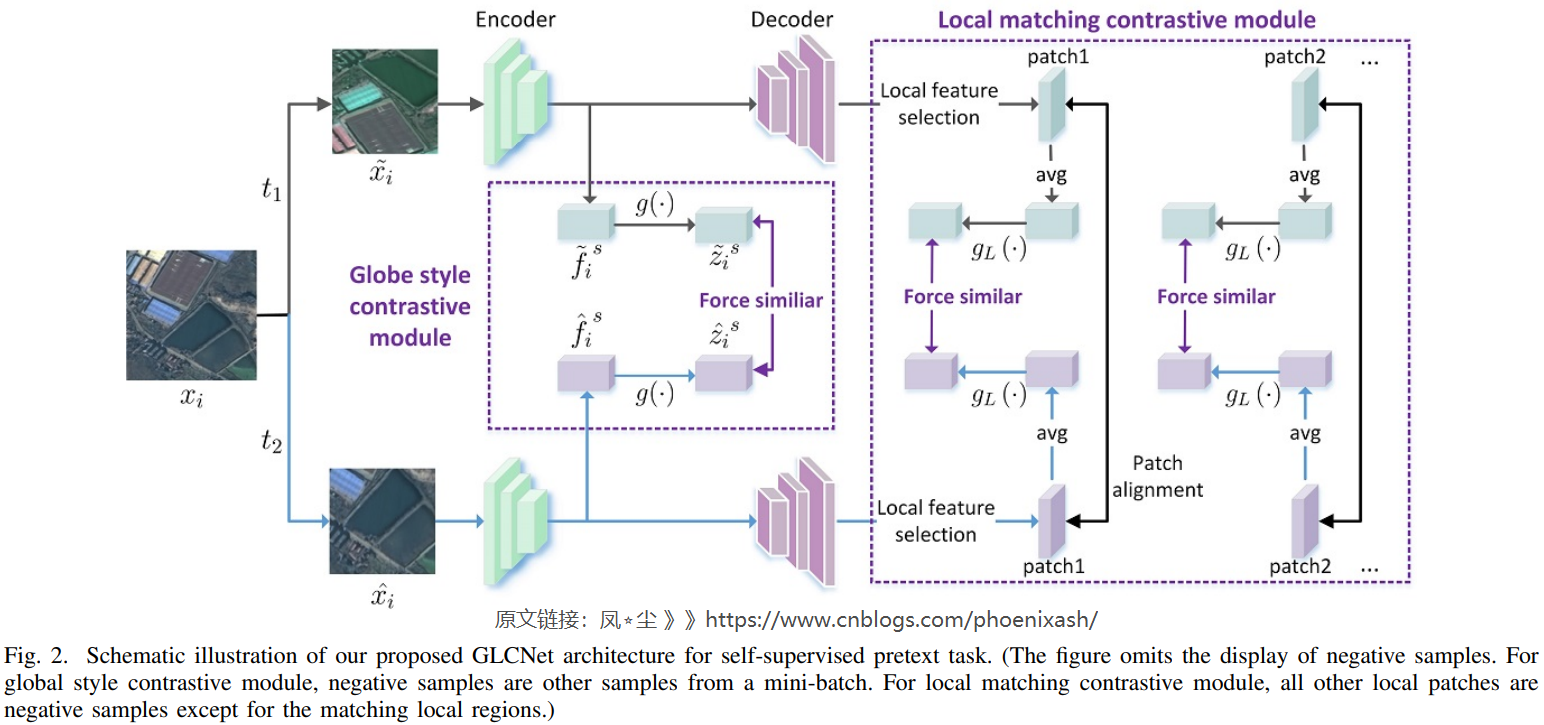### B. Contrastive learning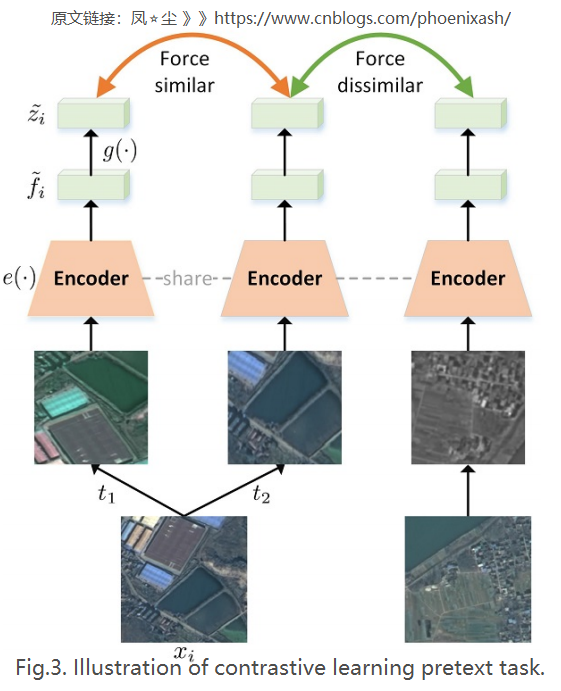​ 1)数据增强:为了鼓励模型能够学习一般的时空不变性特征,如图4所示,我们执行空间变换如随机裁剪,缩放,翻转和旋转来学习空间不变性特征,通过颜色失真、高斯模糊、随机噪声,等模拟时间变换，用于时间不变性特征的学习。具体来说，在给定的样本$x$中，通过数据扩充$t_1$$t_2$，生成两个扩充视图$x$属于和$\hat{x}$，即$x= t_1(x)， \hat{x}= t_2(x)$。 在本工作中，$t_1$表示随机裁剪，然后调整大小到固定分辨率(例如，224x224)，$t_2$表示依次应用几个增强:随机裁剪，然后调整大小到固定分辨率；随机翻转；随机旋转；随机颜色失真；随机高斯模糊；随机噪声和随机灰度。

​ 2)特征提取:利用编码器网络$e(·)$从增广样本实例中提取全局特征：

$\tilde{f}_{i}=\mu\left(e\left(\tilde{x}_{i}\right)\right), \hat{f}_{i}=\mu\left(e\left(\hat{x}_{i}\right)\right)$

​ 3)映射头部分:如式2所示，映射头$g(·)$是一个带有一层隐层(ReLU)的MLP。$g(·)$的存在在SimCLR中被证明是非常有益的，可能是因为它可以让$e(·)$为下游任务构造和保留更多潜在的有用信息。

$\tilde{z}_{i}=g\left(\tilde{f}_{i}\right)=W^{(2)} \sigma\left(W^{(1)} \tilde{f}_{i}\right), \hat{z}_{i}=g\left(\hat{f}_{i}\right)$

​ 4)对比损失:对比损失期望正样本对相似，负样本对不同。具体来说，小批量的N个样品增广为2N个样品。由同一样本扩增的一对样本形成一对正样本，其余2(N-1)个样本为负样本，故将对比损耗$L_C$定义为：

$\mathcal{L}_{C}=\frac{1}{2 N} \sum_{k=1}^{N}\left(\ell\left(\tilde{x_{i}}, \hat{x_{i}}\right)+\ell\left(\hat{x_{i}}, \tilde{x_{i}}\right)\right)$

$\ell\left(\tilde{x}_{i}, \hat{x}_{i}\right)=-\log \frac{\exp \left(\operatorname{sim}\left(\tilde{z}_{i}, \hat{z}_{i}\right) / \tau\right)}{\sum_{x \in \Lambda^{-}} \exp \left(\operatorname{sim}\left(\tilde{z}_{i}, g(f(x))\right) / \tau\right)}$

### C. Global style and Local matching Contrastive learning Network (GLCNet)

​ 1) Global style contrastive learning module: 与已有的实例对比学习类似，全局风格对比学习通过强迫一个样本的不同增强视图与其他样本相似或不同进行学习。不同之处在于，我们使用的是风格特征，而不是实例对比学习中使用的简单的平均池化特征，因为我们认为它更能代表图像的整体特征。黄和Belongie表明CNN提取的channel-wise均值和方差的特征映射可以表示图像的风格特点,所以我们通过计算编码器$e(·)$提取的特征的channel-wise均值和方差，提取全局风格特征向量,定义如下

$f_{s}\left(x_{i}\right)=\operatorname{concat}\left(\mu\left(e\left(x_{i}\right)\right), \sigma\left(e\left(x_{i}\right)\right)\right)$

$\mathcal{L}_{G}=\frac{1}{2 N} \sum_{k=1}^{N}\left(\ell_{g}\left(\tilde{x}_{i}, \hat{x}_{i}\right)+\ell_{g}\left(\hat{x}_{i}, \tilde{x}_{i}\right)\right)$

$\ell_{g}\left(\tilde{x}_{i}, \hat{x}_{i}\right)=-\log \frac{\exp \left(\operatorname{sim}\left(\tilde{z}_{i}^{s}, \hat{z}_{i}^{s}\right) / \tau\right)}{\sum_{x \in \Lambda^{-}} \exp \left(\frac{\operatorname{sim}\left(\tilde{z}_{i}^{s}, g\left(f_{s}(x)\right)\right)}{\tau}\right)}$

​ 2) Local matching contrastive learning:提出局部匹配对比学习模块主要有以下两个原因:第一，单一遥感语义分割图像中的土地覆盖类别极为丰富。仅提取整幅图像的全局特征进行测量和区分，会丢失大量的信息；其次，利用实例对比学习方法获取图像级特征，由于需要像素级的识别，对语义分割可能是次优的。因此，局部匹配对比学习模块被设计用于学习有利于像素级语义的局部区域表示。它由以下主要组件组成：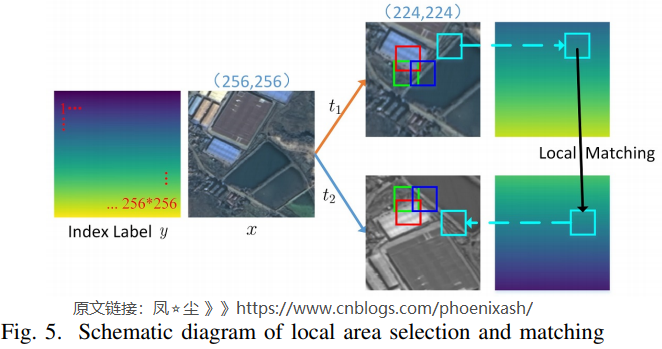a). Local region selection and matching

​ 如图5所示，变换后的两个版本$\tilde{x}$$\hat{x}$来自同一张图像x，即$\tilde{x} = t_1 (x)， \hat{x} = t_2 (x)$，我们从$\tilde{x}$$\hat{x}$中选择并匹配多个局部区域。此外，随机裁剪、翻转、旋转等数据增加操作会导致$\tilde{x}$$\hat{x}$之间的位置不匹配。因此，我们通过引入索引标签来记录像素位置，以确保两个匹配局部区域的中心位置在原始图像中是对应的。具体来说，首先从$\tilde{x}$中随机选择一个大小为$s_p\times s_p$的局部区域，然后根据该局部区域的中心位置索引值确定匹配的相同大小的局部区域在$\hat{x}$中的位置。另外，为了保证不同的局部区域之间不存在过度的重叠，每次选择后都将局部区域排除，保证后续选择的局部区域的中心不落入之前选择的局部区域。以上步骤重复几次，得到多个匹配的局部区域。

b). Local matching feature extraction

​ 局部特征提取步骤如下:首先，从编码器-解码器CNN网络的一对正样本$(\tilde{x}, \hat{x})$中提取特征映射$d (e (\tilde{x}))$$d (e (\hat{x}))$。在本文中，$e(·)$$d(·)$分别对应DeepLabV3+的编码器部分和解码器部分。其次，根据A中的局部区域选择与匹配的思想，从$d (e (\tilde{x}))$$d (e (\hat{x}))$中得到多个匹配局部区域的局部特征图。如果$\tilde{p}_j$$\hat{p}_j$是匹配局部区域的特征映射，其中$\tilde{p}_j$来自$d (e (\tilde{x}))$$\hat{p}_j$来自$d (e (\hat{x}))$，则最终的局部特征向量定义如下:

$\tilde{f}_{L}^{j}=f_{L}\left(\tilde{p_{j}}\right)=\mu\left(\tilde{p_{j}}\right), \hat{f}_{L}^{j}=\mu\left(\hat{p}_{j}\right)$

c). Local matching contrastive loss

​ 局部匹配对比损失通过强制匹配局部区域的特征表示相似和不同局部区域的特征表示不相似来更新一个完整的语义分割码解码器网络。对于一个小批量的N个样本，局部匹配对比度损失定义如下

$\mathcal{L}_{L}=\frac{1}{2 N_{L}} \sum_{j=1}^{N_{L}}\left(\ell_{L}\left(\tilde{p_{j}}, \hat{p}_{j}\right)+\ell_{L}\left(\hat{p}_{j}, \tilde{p}_{j}\right)\right)$

$\begin{gathered}\ell_{L}\left(\tilde{p_{j}}, \hat{p_{j}}\right)=-\log \frac{\exp \left(\operatorname{sim}\left(\tilde{\mu}_{j}, \hat{\mu}_{j}\right) / \tau\right)}{\sum_{p \in \Lambda_{L}^-\exp \left(\frac{\operatorname{sim}\left(\tilde{\mu_{j}}, g_{L}\left(f_{L}(p)\right)\right)}{\tau}\right)}} \\{\tilde{\mu}_{j}=g_{L}\left(\tilde{f}_{L}^{j}\right)=g_{L}\left(f_{L}\left(\tilde{p}_{j}\right)\right), \hat{\mu}_{j}=g_{L}\left(\hat{f}_{L}^{j}\right)}\end{gathered}$

​ 3) Total loss:全局风格对比学习可以捕获全局信息，而局部匹配对比学习可以在局部水平上进行区分，这可能有助于需要像素级识别的语义分割。因此，我们的方法由这两个部分组成，即最终的损失定义如下：

$\mathcal{L} = \lambda\ \cdot\ \mathcal{L}_G\ +\ (1-\lambda)\mathcal{L}_L$

​ 此外，我们提供了算法1来详细描述我们提出的GLCNet。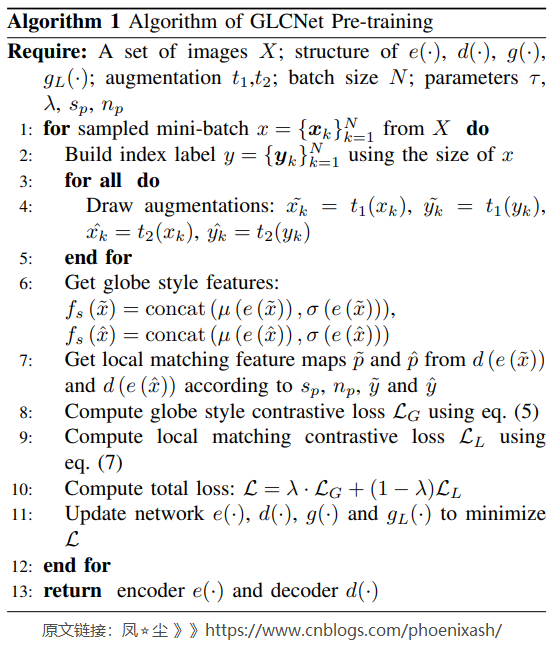posted @ 2021-10-06 15:11  凤☆尘  阅读(195)  评论(0编辑  收藏  举报